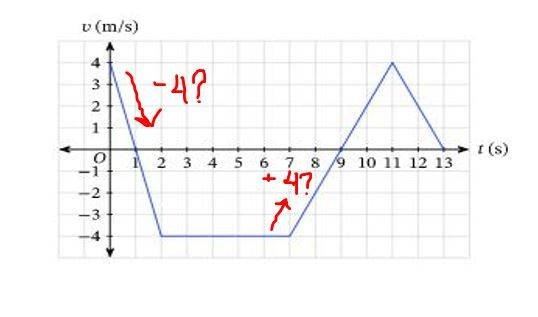# Understanding a Velocity-Time Graph

Summary:: I think we are still in the earlier parts of Physics and I am confused at how "values" work for a velocity-time graph. We are using the formulas to solve an area of a triangle and rectangle to find the total displacement. If a diagonal line begins from above and continue to go down, that denotes a negative velocity but in solving for the height of a triangle, will it also be negative?For the area of triangle, we use " 1/2(b)(h) ". For the first triangle will its height be a -4 in solving, as the line is going down therefore it is decelerating, or should I use a positive 4? How about the two other triangles in the trapezoid? How about the rectangle, will its height be -4?
We were asked to solve the displacement within the time frame of (0,9) so the triangle beyond that is not a concern for me at the moment.

Homework Helper
Gold Member
2022 Award
What is displacement?

What is the relationship between velocity and displacement?

Can you sketch a displacement vs time graph from the velocity vs time graph?

What is displacement?

What is the relationship between velocity and displacement?

Can you sketch a displacement vs time graph from the velocity vs time graph?
Based on my understanding:

Displacement is a scalar measurement that quantifies how far we have gone from an original point/place/position relative to direction as opposed to Distance which simply measures, well, total "distance."

Similarly, velocity takes into account direction. And its relationship with displacement tells us how fast you have gone in any specified direction relative to where you began.

That is why we can have negative velocity and displacement as that would mean you went the "opposite direction"; backward instead of forward.

I think yes, once you've found the displacement.

I may be wrong but please correct me, thank you very much.

Homework Helper
Gold Member
2022 Award
Based on my understanding:

Displacement is a scalar measurement that quantifies how far we have gone from an original point/place/position relative to direction as opposed to Distance which simply measures, well, total "distance."

Similarly, velocity takes into account direction. And its relationship with displacement tells us how fast you have gone in any specified direction relative to where you began.

That is why we can have negative velocity and displacement as that would mean you went the "opposite direction"; backward instead of forward.

I think yes, once you've found the displacement.

I may be wrong but please correct me, thank you very much.
Displacement is a vector quantity. Distance is a scalar.

Velocity is the rate of change of displacement.

The displacement at ##1s, 2s, 3s##.

Pardon me, I mixed them up.

In solving of the triangle, should my solution be 1/2(1)(-4)?

Homework Helper
Gold Member
2022 Award
In solving of the triangle, should my solution be 1/2(1)(-4)?

The displacement at ##1s, 2s, 3s##.

Why don't you calculate the displacement at ##1s, 2s, 3s##?

If in doubt calculate!

Why don't you calculate the displacement at ##1s, 2s, 3s##?

If in doubt calculate!
Would the displacement at 1s be 2 cm?

Homework Helper
Gold Member
2022 Award
Would the displacement at 1s be 2 cm?
Why ##cm##?

Why ##cm##?
I'm really sorry, I meant 2m. Would that be correct?

Homework Helper
Gold Member
2022 Award
I'm really sorry, I meant 2m. Would that be correct?
Yes.

And at 2s, the displacement would be 0m because of 2m + (-2m)?

•PeroK
Homework Helper
Gold Member
2022 Award
And at 2s, the displacement would be 0m because of 2m + (-2m)?
Yes. In summary:

When the velocity is positive, the graph is above the ##t## axis and area represents a positive displacement. Regardless of the sign of the acceleration.

When the velocity is negative, the graph is below the ##t## axis and area represents a negative displacement.

Note: if you have studied calculus, the integral takes care of this for you. If you integrate a velocity vs time function, then the integral automatically calculates anything below the ##t## axis as negative.

If you calculate the areas by hand, you have to put in the negative signs for anything below the ##t## axis.

Note that that signs on your original diagram were the wrong way round. As you now know.

Yes. In summary:

When the velocity is positive, the graph is above the ##t## axis and area represents a positive displacement. Regardless of the sign of the acceleration.

When the velocity is negative, the graph is below the ##t## axis and area represents a negative displacement.

Note: if you have studied calculus, the integral takes care of this for you. If you integrate a velocity vs time function, then the integral automatically calculates anything below the ##t## axis as negative.

If you calculate the areas by hand, you have to put in the negative signs for anything below the ##t## axis.

Note that that signs on your original diagram were the wrong way round. As you now know.
Thank you very much! This helps me greatly. And I have taken Calculus, that would make sense. Thank you again and have a nice day :)

Oh, and I wouldn't want to get complacent hahaha, upon calculating: the total displacement in 9s would be -24m, correct?

Mentor
Oh, and I wouldn't want to get complacent hahaha, upon calculating: the total displacement in 9s would be -24m, correct?
Correct.

If you make separate triangles for times where the velocity is positive and regions where it is negative then it's easier to keep track of the signs.

In some cases symmetry arguments work as well. Between t=0 and t=2 for example: As you see both triangles cancel each other to get 0 displacement. Knowing the area of the triangles isn't important as long as you know the areas are the same (but on opposite sides of the x axis).